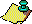Contents Next: Ergodic Results Up: Lyapunov Exponents Previous: Lyapunov Exponents

There are infinitely many initial points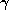in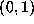with this Lyapunov exponent. For example, all the numbers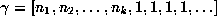, that is, all the numbers whose continued fractions ultimately end in 1's, have Lyapunov exponent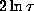. These are the so-called noble numbers , noticed for their resistance to chaos, and we see here that they all share the (still positive) minimum possible Lyapunov exponent under the Gauss map.

One might ask if there are non-noble numbers with this Lyapunov exponent, and indeed I think the answer is yes. The trick to this is constructing numbers with lots of 1's in the continued fraction. For example,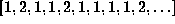, where there are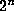1's in between the 2's, might work. I do not know of a good characterization of the set of all such noble-equivalent or `nearly noble' numbers.Contents Next: Ergodic Results Up: Lyapunov Exponents Previous: Lyapunov Exponents